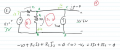# What's the value of the voltage supply in this circuit?

#### Rockyalife

Joined Oct 20, 2019
1
Hi,
I've been trying to find out the answer to this question. I know that the final answer is 3V but I can't seem to prove that.

I always get confused when there's two or more supplies on the circuit.I've already tried the kirchhoff laws but I've never reached to the same result.
Can someone give me a hand and let me know if I at least pointed the directions of the current properly? And tell me the best approach that I should try next?

Thank you!#### WBahn

Joined Mar 31, 2012
26,156

-Vs + R1·Is + R2·I2 = 0

What is R2·I2 represent?

If you want it to represent the voltage drop across R2, then don't you need to use the current flowing in R2? Is the current flowing in R2 equal to I2? Think carefully.

#### KeithWalker

Joined Jul 10, 2017
1,222
Hi,
I've been trying to find out the answer to this question. I know that the final answer is 3V but I can't seem to prove that.

I always get confused when there's two or more supplies on the circuit.I've already tried the kirchhoff laws but I've never reached to the same result.
Can someone give me a hand and let me know if I at least pointed the directions of the current properly? And tell me the best approach that I should try next?

Thank you!

View attachment 188377
Forget Kirchoff. Use some simple logic. You know there is 6 volts across the second resistor. Therefore you can calculate the total current through the first resistor. From that you can deduce the voltage across it.

#### WBahn

Joined Mar 31, 2012
26,156
Forget Kirchoff. Use some simple logic. You know there is 6 volts across the second resistor. Therefore you can calculate the total current through the first resistor. From that you can deduce the voltage across it.
But if he can't get a solution using Kirchhoff's laws (or any of the other basic techniques), then it would behoove him to spend the time necessary to figure out (and understand) how to apply those techniques to this circuit. Otherwise he is just kicking the can down the road and digging himself a deeper hole that will only be more difficult to get back out of later.

•SamR